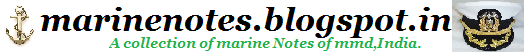Home » » Centrifugal Pump Fundamentals 2

# Centrifugal Pump Fundamentals 2

 STATIC DISCHARGE HEAD is the vertical distance in feet between the pump centerline and the point of free discharge or the surface of the liquid in the discharge tank. TOTAL STATIC HEAD is the vertical distance in feet between the free level of the source of supply and the point of free discharge or the free surface of the discharge liquid. FRICTION HEAD (hf) is the head required to overcome the resistance to flow in the pipe and fittings. It is dependent upon the size, condition and type of pipe, number and type of pipe fittings, flow rate, and nature of the liquid. Frictional tables are included in Water Data. VELOCITY HEAD (hv) is the energy of a liquid as a result of its motion at some velocity V. It is the equivalent head in feet through which the water would have to fall to acquire the same velocity, or in other words, the head necessary to accelerate the water. Velocity head can be calculated from the following formula:The velocity head is usually insignificant and can be ignored in most high head systems. However, it can be a large factor and must be considered in low head systems. PRESSURE HEAD must be considered when a pumping system either begins or terminates in a tank which is under some pressure other than atmospheric. The pressure in such a tank must first be converted to feet of liquid. A vacuum in the suction tank or a positive pressure in the discharge tank must be added to the system head, whereas a positive pressure in the suction tank or vacuum in the dis-charge tank would be subtracted. The following is a handy formula for converting inches of mercury vacuum into feet of liquid.The above forms of head, namely static, friction, velocity, and pressure, are combined to make up the total system head at any particular flow rate. Following are definitions of these combined or "Dynamic" head terms as they apply to the pump. TOTAL DYNAMIC SUCTION LIFT (hs) is the static suction lift minus the velocity head at the pump suction flange plus the total friction head in the suction line. The total dynamic suction lift, as determined on pump test, is the reading of a gauge on the suction flange, converted to feet of liquid and corrected to the pump centerline*, minus the velocity head at the point of gauge attachment. TOTAL DYNAMIC SUCTION HEAD (hs) is the static suction head plus the velocity head at the pump suction flange minus the total friction head in the suction line. The total dynamic suction head, as determined on pump test, is the reading of the gauge on the suction flange, converted to feet of liquid and corrected to the pump centerline*, plus the velocity head at the point of gauge attachment. TOTAL DYNAMIC DISCHARGE HEAD (hd) is the static discharge head plus the velocity head at the pump discharge flange plus the total friction head in the discharge line. The total dynamic discharge head, as determined on pump test, is the reading of a gauge at the discharge flange, converted to feet of liquid and corrected to the pump centerline*, plus the velocity head at the point of gauge attachment. TOTAL HEAD (H) or TOTAL Dynamic HEAD (TDH) is the total dynamic discharge head minus the total dynamic suction head or TDH = hd + hs (with a suction lift) TDH = hd - hs (with a suction head)